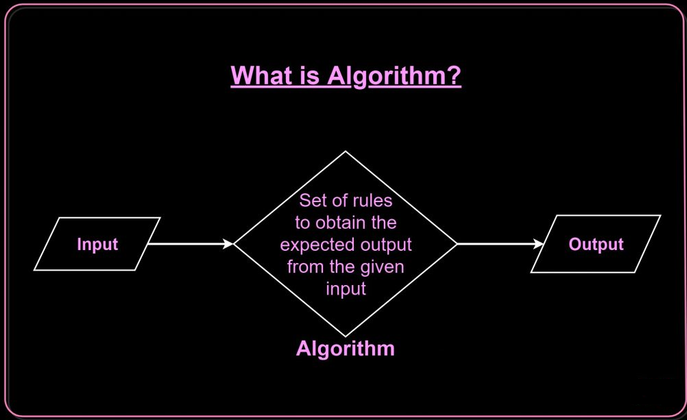## CodeNewbie Community is a community of 8,488 amazing newbies# What is Algorithm in C Programming

In this tutorial, we will study What is an Algorithm in C Programming and its example, the qualities required in the algorithm, and the different types of algorithms available, and algorithm complexity. So let us start.

## What is an algorithm

An algorithm can be seen as a series of steps for solving a problem. The algorithm lays the foundation for writing a program. To solve any problem we have to go through different steps which are defined as a group. The steps to be followed are organized and are written in simple language.

Example:- If we want to add A and B to obtain C. Then we have to follow the following steps.

1. Start
2. Value of A
3. Value of B
5. The sum of A and B is placed in the place of C place
6. Print the value of C
7. Process End.

For adding 2 numbers we can perform different steps in the same serial order

## What are the qualities an algorithm should have?

1. Input and output should be defined precisely.
2. Every step in the algorithm should be clear and unambiguous.
3. Algorithms should be most effective among various ways to solve a problem.
4. An algorithm shouldn't have a computer code. Instead, the algorithm should be written in such a way that it can be used in different programming languages.

## What are the different types of algorithms?

• Recursive algorithm
• Dynamic programming algorithm
• Backtracking algorithm
• Divide and conquer algorithm
• Greedy algorithm
• Brute force algorithm
• Randomized algorithm

## What is an Algorithm Complexity and How to find it?

An algorithm is defined as complex based on the amount of Space and Time it consumes. Hence the Complexity of an algorithm refers to the measure of the Time that it will need to execute and get the expected output, and Space it will need to store all the data (input, temporary data, and output). Hence these two factors define the efficiency of an algorithm.

The two factors of Algorithm Complexity are:

1. Time Factor: The time factor is measured by counting the number of key operations such as comparisons in the sorting algorithm.
2. Space Factor: Space factor is measured by counting the maximum memory space required by the algorithm.

## What are the advantages of an algorithm?

• It uses a definite procedure
• It does not depend upon any programming language
• It is easy to understand by any person
• Each step in the algorithm has its own logical sequence
• It is easy to debug
• The problem is broken down into smaller pieces and is much easier to solve

## What are the disadvantages of an algorithm?

• They can be rigid sometimes
• The scope of the problem can change and the working algorithm may not be any longer flexible
• It is time-consuming
• The big tasks are difficult to be put in an algorithm
• It is difficult to show branching and looping in the algorithm